• 启发函数
千次阅读
2017-12-11 09:01:19

这个应该是搜索部分最有实用意义的一部分。给定一个搜索问题，如何确定一个启发函数，这无意是最具指导意义的。

考虑一个实际问题，8数码问题：在3×3的格子上有8个棋子，1-8，随机摆放在棋盘的四周格子中，其中中间的一格没有棋子，要通过移动，把这8个棋子变成按1-8的顺序排列的棋子。并且空格在0,0位置。

这里有两个启发函数
h1 = 不在位的棋子数，如果某状态所有的棋子都不在正确的位置，那么h1=8.可以知道这是一致性的启发函数。

h2=所有棋子到其目标位置的距离和，因为棋子不能斜着移动，这里的距离是指水平和竖直距离的和。这个函数也是一致的。

这两个启发函数，到底哪个好呢？一种刻画启发式函数的方法是有效分支因子b* , 对于某个问题，如果A*算法生成的总结点数为N，解的深度为d。那么b*就是深度为d的标准搜索树为了能够包括这N+1个节点所必需的分支因子。即
N+1 = 1+ b* + pow(b*,2) + pow(b*,3) + … + pow(b*,d)

如果A*算法用52个节点在第5层找到了解，那么b*就是1.92. 这个因子在实际问题中通常比较稳定，因此可以在小规模范围内估计这个b*，然后在实际问题中采用最小的b*来处理问题。

实际实验证明h2要比h1要好。

其实从另一个方面，如果h2/h1,是两个一致启发函数，并且h2(n)>h1(n) , 那么h2是比h1更好的启发函数。这是因为A*算法总是搜索所有的f(n) < C* 的节点。 而f(n) = g(n) + h(n) ,  h(n)越大，那么对应的g(n)就越小，满足f(n) < C*的节点就越少。

那么如何设计h2这个启发函数呢？计算机能否设计出这样的函数呢？

h1/h2估计剩余路径的长度，如果我们改变游戏规则，是棋子可以随便移动，而不是只能移动到起相邻的空格上，那么h1将给出最短解的确切步数。如果棋子每次可以横向或者纵向移动一格，而不管这个位置原来是不是有棋子，那么h2将给出最短解的确切步数。这里，减少了行动限制问题的问题成为松弛问题。松弛状态问题是原有状态空间的超图，因为减少限制导致图的边增加。

由于松弛问题增加了状态空间的边，原有问题的最优解是松弛问题的“最优”解，但是松弛问题可能存在更好的解。所以一个松弛问题的最优解代价是原问题的可采纳的启发式。更进一步，由于得出的启发式是松弛问题的确切代价，那么他一定遵守三角不等式，因而是一致的。

考虑上面的8数码问题，其行动可描述如下：棋子可以从A移到B，如果A与B水平或竖直相邻，并且B是空的。

如果去掉其中一个或者两个条件，生成三个松弛问题：

1.棋子可以从A移到B，如果A和B是相邻的。
2.棋子可以从A移到B，如果B是空的
3.棋子可以从A移到B。

由1，我们可以得到h2； 由3，可以得到h1。而由二，我们可以得到第三个启发函数。

如果我们得到一些列的启发函数，h1,h2,h3,但是找不大一个明显好的启发函数，那可以用h(n)=max(h1(n),h2(n),…,hm(n)) 来作为启发函数，而这个启发函数比任何一个单个的启发函数都要好。

可以证明h(n)是一致的，如果每个hi(n)都是一致的。
这个可以通过反证法得到，假设h(n)=hi(n) ,  h(n’)=hj(n’) , 现在要证明hi(n)<=c(n,a,n’) + hj(n’)
假设hi(n)  > c(n,a,n’) +hj(n’)  , 那么因为hj(n’) > hi(n’),  可以得到hi(n) > c(n,a,n’) + hi(n’) . 这与hi是一致启发函数相矛盾。因此，max(h1,h2,h3,…hm)是一致的启发函数。因为这函数比所有的启发函数都要大，因此她比所有的启发函数的都要好。

另外从子问题出发，也可以设计处可采纳的启发式。依旧考虑上面的8数码问题，如果只考虑将棋子1，2，3，4移动到正确的位置上，显然这个子问题的最优解的代价是完整问题的解代价的一个下界。在某些情况下，比上面提到的h2 要更好。

模式数据库的想法就是对每个可能的子问题实例存储解代价，最为一个启发函数。而1，2，3，4的选择是随机的，我们也可以选择自问题，2，3，4，5或者5，6，7，8，这样对于每个子问题。我们都可以构造一个启发函数，然后取MAX(hi)得到一个较好的启发函数。通过这样的方式，可以构造出更加精确的启发函数。

文章出处：http://www.bagualu.net/wordpress/archives/3431机器学习
更多相关内容
• 以八数码难题实例的启发式搜索,分析了估价函数 f( n)中不同的启发函数 h( n)对搜索过程的影响,比较了3种不同 h(n)的搜索效率。指出了选择最佳启发函数 h*( n)的原则,讨论了八数码难题启发函数思路的通用性,并对A*...
• 启发函数 (Heuristic Function) 盲目搜索会浪费很多时间和空间, 所以我们在路径搜索时, 会首先选择最有希望的节点, 这种搜索称之为"启发式搜索 (Heuristic Search)" 如何来界定"最有希望"? 我们需要通过启发函数 ...

启发函数 (Heuristic Function)

盲目搜索会浪费很多时间和空间, 所以我们在路径搜索时, 会首先选择最有希望的节点, 这种搜索称之为 "启发式搜索 (Heuristic Search)"

如何来界定"最有希望"? 我们需要通过 启发函数 (Heuristic Function) 计算得到.

对于网格地图来说, 如果只能四方向(上下左右)移动, 曼哈顿距离(Manhattan distance) 是最合适的启发函数.

function Manhattan(node) =
dx = abs(node.x - goal.x)
dy = abs(node.y - goal.y)
// 在最简单的情况下, D 可以取 1, 返回值即 dx + dy
return D * (dx + dy)

如果网格地图可以八方向(包括斜对角)移动, 使用 切比雪夫距离(Chebyshev distance) 作为启发函数比较合适.

function Chebyshev(node) =
dx = abs(node.x - goal.x)
dy = abs(node.y - goal.y)
// max(dx, dy) 保证了斜对角的距离计算
return D * max(dx, dy)

如果地图中允许任意方向移动, 不太建议使用网格 (Grid) 来描述地图, 可以考虑使用 路点 (Way Points) 或者 导航网格 (Navigation Meshes) , 此时使用 欧式距离(Euclidean distance) 来作为启发函数比较合适.

function heuristic(node) =
dx = abs(node.x - goal.x)
dy = abs(node.y - goal.y)
// 在最简单的情况下, D 可以取 1, 返回值即 sqrt(dx * dx + dy * dy)
return D * sqrt(dx * dx + dy * dy)

欧式距离因为有一个 sqrt() 运算, 计算开销会增大, 所以可以使用 Octile 距离 来优化(不知道怎么翻译), Octile 的核心思想就是假定只能做 45 度角转弯.

function heuristic(node) =
dx = abs(node.x - goal.x)
dy = abs(node.y - goal.y)
k = sqrt(2) - 1
return max(dx, dy) + k * min(dx, dy)

下图是一个启发函数的简单示意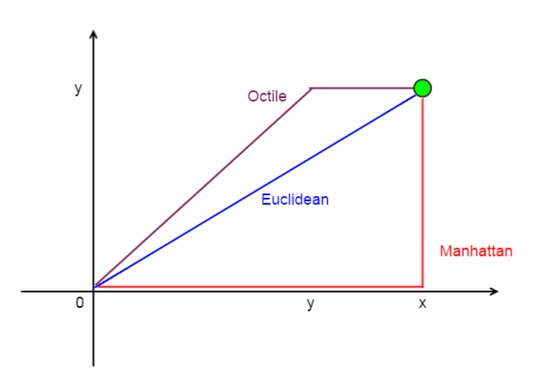## Dijkstra

Dijkstra 算法 是由计算机大牛 Dijkstra 在1956年提出来的, 它是一个"精确"的寻路算法, 能保证发现节点之间的最短路径, 本质是一个 广度优先搜索 .

• g(n): 从起始点到当前点 n 的开销, 在网格地图中一般就是步长.
• 将起始点 S 加入 open-list.
• 从当前的 open-list 中选取 g(n) 最优(最小)的节点 n, 加入 closed-list
• 遍历 n 的后继节点 ns
• 如果 ns 是新节点, 加入 open-list
• 如果 ns 已经在 open-list 中, 并且当前 h(ns) 更优, 则更新 ns 并修改 parent
• 迭代, 直到找打目标节点 D, 回溯得到路径

Dijkstra 算法的效率并不高, 时间复杂度为 O(n^2), 但是它保证了寻路结果是最短距离.

## Best-First-Search

Best-first Search 最佳优先搜索, 简称为 BFS.

BFS 根据启发式函数的推断, 每次迭代最优的节点, 直到寻找到目标节点. 一个简单的算法流程如下:

• h(n) 代表从当前点 n 到目标点 S 的估算开销, 即启发函数.
• 将起始点 S 加入 open-list.
• 从当前的 open-list 中选取 h(n) 最优(最小)的节点 n, 加入 closed-list
• 遍历 n 的后继节点 ns
• 如果 ns 是新节点, 加入 open-list
• 如果 ns 已经在 open-list 中, 并且当前 h(ns) 更优, 则更新 ns 并修改 parent
• 迭代, 直到找打目标节点 D, 回溯得到路径

如果不使用 open-list, 直接每次都寻找 n 的后继节点中最优的节点, BFS 则退化为贪心最佳优先搜索 (Greedy BFS).

不管是 BFS 还是 Greedy-BFS, 本质上都还是寻找 局部最优 , 虽然效率会比 Dijkstra 高很多, 但最终得到的解并不一定是全局最优(最短距离), 在地图中有障碍物时尤为明显.

## A-Star

A-Star 俗称 A* , 是应用最广的寻路算法, 在很多场景下都适用. A* 兼顾了 Dijkstra 的准确度和 BFS 的效率.

• f(n) = g(n) + h(n) , g(n) 和 h(n) 的定义同上
• 当 g(n) 退化为 0, 只计算 h(n) 时, A* 即退化为 BFS.
• 当 h(n) 退化为 0, 只计算 g(n) 时, A* 即退化为 Dijkstra 算法.
• 将起始点 S 加入 open-list.
• 从当前的 open-list 中选取 f(n) 最优(最小)的节点 n, 加入 close-list
• 遍历 n 的后继节点 ns
• 如果 ns 是新节点, 加入 open-list
• 如果 ns 已经在 open-list 中, 并且当前 f(ns) 更优, 则更新 ns 并修改 parent
• 迭代, 直到找打目标节点 D.

A* 算法在从起始点到目标点的过程中, 尝试平衡 g(n) 和 h(n), 目的是找到最小(最优)的 f(n) .

## A-Star 算法衍生

如果在每一步迭代扩展中, 都去除掉质量比较差的节点, 即选取 有限数量的后继节点 , 这样减少了空间开销, 并提高了时间效率. 但是缺点可能会丢弃潜在的最佳方案. 这就是 集束搜索(Beam Search) , 本质上还是局部最优的贪心算法.

当距离起点越近, 快速前行更重要; 当距离目标点越近, 到达目标更重要. 基于这个原则, 可以引入权重因子, A* 可以演进为选取 f(n) = g(n) + w(n) * h(n) 最优的点, w(n) 表示在点 n 的权重. w(n) 随着当距离目标点越近而减小. 这是 动态加权 A* (Dynamic Weighting A*) .

当从出发点和目标点同时开始做双向搜索, 可以利用并行计算能力, 更快的获得计算结果, 这是 双向搜索 (Bidirectional Search) . 此时, 向前搜索 f(n) = g(start, n) + h(n, goal), 向后搜索 f(m) = g(m, goal) + h(start, m), 所以双向搜索最终可以归结为选取 g(start, n) + h(m, n) + g(m, goal) 最优的一对点(m, n).

A* 在静态地图中表现很棒, 但是在动态环境中(例如随机的障碍物), 却不太合适, 一个基于 A* 算法的 D* 算法(D-Star, Dynamic A*) 能很好的适应这样的动态环境. D* 算法中, 没有 g(n), f(n) 退化为 h(n), 但是每一步 h(n) 的计算有所不同: h(n) = h(n-1) + h(n-1, n) , c(n-1, n) 表示节点 n-1 到节点 n 的开销.

展开全文算法 自动驾驶
• 作业笔记lgm： 估价函数 f(x)=deep(搜索深度)+distance(与目标状态距离)f...1.主函数：实现八数码的求解：start,end,heuristic(启发函数) def eight_digit(start, end, heuristic=None): # 初始化结点 node = Node()

作业笔记lgm：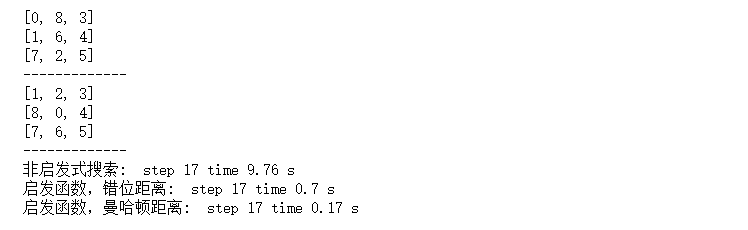#### 估价函数

f ( x ) = d e e p ( 搜 索 深 度 ) + d i s t a n c e ( 与 目 标 状 态 距 离 ) f(x)=deep(搜索深度)+distance(与目标状态距离)
distance： 曼哈顿距离，棋盘错位距离

#### 1.主函数：实现八数码的求解：start,end,heuristic(启发函数)

def eight_digit(start, end, heuristic=None):
# 初始化结点
node = Node()
node.state = start
node.distance =  distance(node.state,end,heuristic)
node.deep = 0
node.value = node.distance + node.deep

# 初始化openList，closedList
openList = []
closedList = []

openList.append(node)

# 是否寻找到目标状态
complete = False

while openList:
# 是否使用启发函数
if heuristic:
openList.sort(key=lambda x: x.value)
node = openList.pop(0)
closedList.append(node)

# 四个移动的方向
directions = ['up','down','left','right']
for direction in directions:
move_node = move(node, end, heuristic, direction)
if move_node:
if not node_in_list(move_node,openList):
if not node_in_list(move_node,closedList):
openList.append(move_node)
# 到达目标状态
if move_node.distance==0:
closedList.append(move_node)
complete = True
break
if complete:
break

# 提取开始状态到目标状态的所经历的状态
node = closedList.pop()
process_state = []
while node:
process_state.insert(0,(node.direction,node.state))
node = node.parent

return process_state


#### 2.结点Node数据类型

class Node:
state = None
distance = None
deep = None
value = None
parent = None
direction = None


#### 3.距离

def distance(state:list, end:list, heuristic='Manhattan'):
# 错位距离
distance = 0
if heuristic == 'misplace':
for i in range(9):
if state[i]!=end[i]:
distance += 1
return distance

# 曼哈顿距离
distance = 0
for num in range(1,9):
index = state.index(num) + 1
sta_row = index//3 if index%3==0 else index//3 + 1
sta_col = 3 if index%3==0 else index%3
index = end.index(num) + 1
end_row = index//3 if index%3==0 else index//3 + 1
end_col = 3 if index%3==0 else index%3
distance += abs(sta_col-end_col) + abs(sta_row-end_row)
return distance


#### 4.数码移动

# 空格移动
def move(last_node:Node, end, heuristic, direction=None):
if not direction: return None
node = deepcopy(last_node)
index = node.state.index(0)

# 判断是否能完成指定方向的移动
if direction=='up' and 0<=index<=2: return None
if direction=='down' and 6<=index<=8: return None
if direction=='left' and index in [0,3,6]: return None
if direction=='right' and index in [2,5,8]: return None

# 移动
if direction == 'up': step = -3
elif direction == 'down': step = 3
elif direction == 'left': step = -1
elif direction == 'right': step = 1
node.direction = direction
node.state[index] = node.state[index + step]
node.state[index+step] = 0
node.distance = distance(node.state, end, heuristic)
node.deep = node.deep + 1
node.value = node.distance + node.deep
node.parent = last_node
return node


#### 5.判断结点是否在list中

def node_in_list(node:Node, nodeList:list):
state_list = [n.state for n in nodeList]
if node.state in state_list:
return True
else:
return False


#### 6.测试

def main(start,end):
show(start)
show(end)
start_time = time.time()
result_M = eight_digit(start,end)
end_time = time.time()
spend_time0 = round(end_time - start_time, 2)

start_time = time.time()
result_M = eight_digit(start,end,'misplace')
end_time = time.time()
spend_time1 = round(end_time - start_time, 2)

start_time = time.time()
result_ = eight_digit(start,end,'Manhattan')
end_time = time.time()
spend_time2 = round(end_time - start_time, 2)

print('非启发式搜索: ', 'step',len(result_M),'time', spend_time0,'s')
print('启发函数，错位距离: ','step',len(result_), 'time',spend_time1,'s')
print('启发函数，曼哈顿距离: ','step',len(result_M), 'time', spend_time2,'s')
start = [0,8,3,1,6,4,7,2,5]
end = [1,2,3,8,0,4,7,6,5]
main(start,end)展开全文• 1、启发函数——曼哈顿距离等 2、权重系数——动态加权等 3、搜索邻域——基于8邻域搜索改进 4、搜索策略——双向搜索、JPS策略等 5、路径平滑处理——贝塞尔曲线、B样条曲线等 一、启发函数（理论） A星算法评价...

基于Python语言对A星算法进行优化：(视频中会拿python与matlab作对比)

源码地址：https://github.com/Grizi-ju/ros_program/blob/main/path_planning/Astar.py
B站详解视频：https://www.bilibili.com/video/BV1FL4y1M7PM?spm_id_from=333.999.0.0
基于开源算法：https://github.com/Grizi-ju/PythonRobotics/tree/master/PathPlanning/AStar
（个人认为，用哪种语言不重要，重要的是改进点及改进思路）

将从以下5个点进行改进：
1、启发函数——曼哈顿距离等
2、权重系数——动态加权等
3、搜索邻域——基于8邻域搜索改进
4、搜索策略——双向搜索、JPS策略等
5、路径平滑处理——贝塞尔曲线、B样条曲线等

### 一、基础代码详解

本人已经做了详细的中文注释，代码地址：https://github.com/Grizi-ju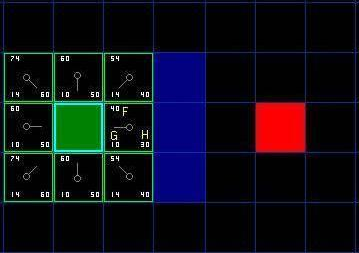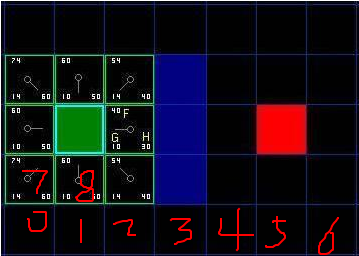算法预处理：
1、将地图栅格化，每一个正方形格子的中央成为节点（索引index）
2、确定起始点和目标点
3、定义open_set列表与closed_set列表，open_set中存放待考察的节点，closed_set中存放已经考察过的节点
4、初始时，定义起点为父节点，存入closed_set
5、父节点周围共有8个节点，定义为子节点，并存入open_set中，成为待考察对象

### 二、启发函数改进（理论）

A星算法评价函数为f(n)=g(n)+h(n)，其中h(n)为启发函数，启发函数的改进对算法行为影响很大
启发函数的作用：指引正确的扩展方向
其中：
f(n) 是节点 n的评价函数
g(n)是在状态空间中从初始节点到节点 n的实际代价
h(n)是从节点n到目标节点的最佳路径的估计代价。
g(n)是已知的，所以在这里主要是 h(n) 体现了搜索的启发信息。换句话说，g(n)代表了搜索的广度的优先趋势。

#### 0、Dijkstra

如果h(n)=0,那么只有g(n)实际上是有用的,这时A*算法退化成迪杰斯特拉算法,它能保证一定可以找到一条最优路径

Dijkstra和贪心算法的缺点：
1.Dijkstra算法很好地找到了最短路径，但它浪费了时间去探索那些没有前途的方向。
2.贪婪的最好的第一次搜索在有希望的方向上探索，但它可能找不到最短的路径。

A*算法结合了这两种方法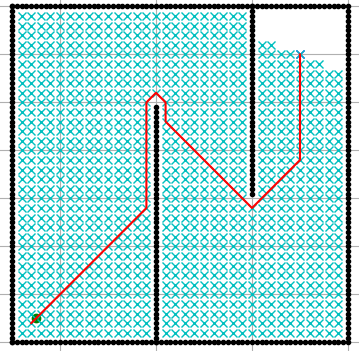#### 1、曼哈顿距离

标准的启发函数是曼哈顿距离（Manhattan distance）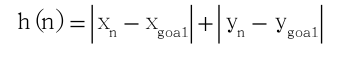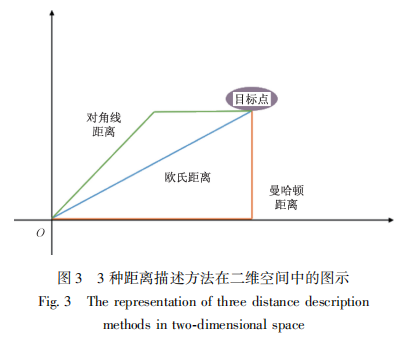h = np.abs(n1.x - n2.x) + np.abs(n1.y - n2.y)     #  Manhattan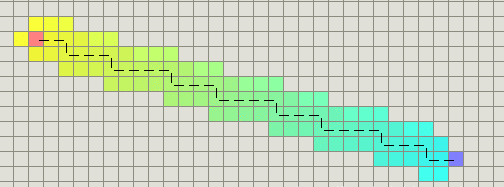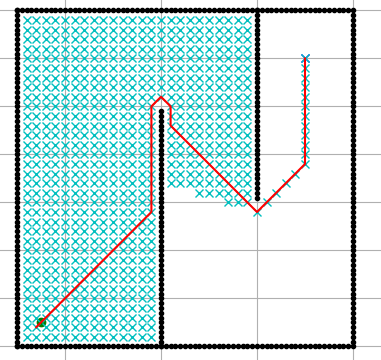#### 2、欧几里得距离（欧氏距离）

如果单位可以沿着任意角度移动（而不是网格方向），那么也许应该使用直线距离：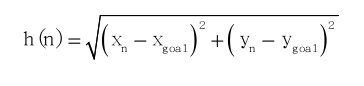h = math.hypot(n1.x - n2.x, n1.y - n2.y)       #  Euclidean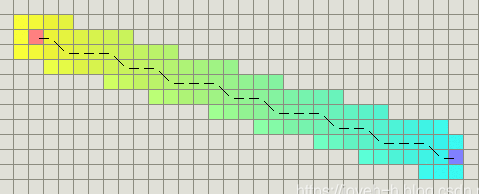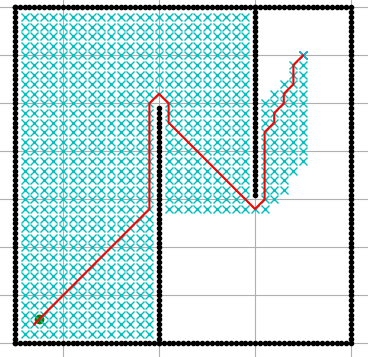#### 3、对角线距离（切比雪夫距离）

如果在地图中允许对角运动，那么需要一个不同的启发函数

dx = np.abs(n1.x - n2.x)                        #  Diagnol distance
dy = np.abs(n1.y - n2.y)
min_xy = min(dx,dy)
h = dx + dy + (math.sqrt(2) - 2) * min_xy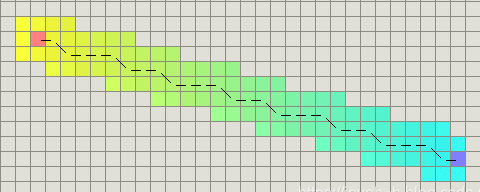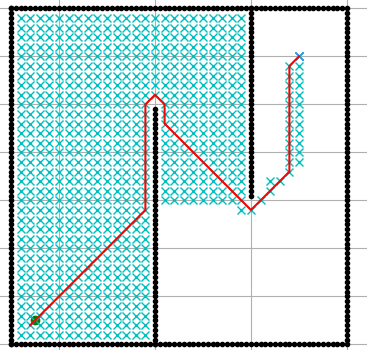参考论文：《一种面向非结构化环境的改进跳点搜索路径规划算法》等

展开全文算法 matlab python
• consistent heuristic 启发的一致性 admissible heuristic 可接受启发 https://blog.csdn.net/haolexiao/article/details/70302848 https://www.jianshu.com/p/c728bb269116 ...
• 作为我出山的第一篇日志，怎么也得写篇对得起我身份和地位的文章吧? 先容我吐槽一下不小心发的贴图...举个栗子，如果你身处迷宫，但你知道出口的方向，那么你应该会尝试往出口方向前进，那么，第一种启发函数就被确...
• 八数码问题 八数码问题（重排九宫...A*算法（启发式搜索） 在一个搜索图中，每下一步我们需要从所有叶节点中选择一个节点扩展。为了尽快找到从初始节点到目标节点的一条耗散值比较小的路径，我们希望所选择的节点尽...c++ 算法 数据结构
• ## A*算法与启发函数

千次阅读 2018-04-22 00:19:48
A*算法为什么叫这个名这个从wiki上看来的，一开始是57年提出的Dijkstra算法，然后64年Nils Nilsson提出了A1算法，是一个启发式搜索算法，而后又被改进成为A2算法，直到68年，被Peter E. Hart改进成为A*算法，为什么...A*算法
• 首先我们回顾一下这几种决策树构造时使用的准则。 ID3-最大信息增益
• 通过分析传统方法的不足，提出用于反演消解的启发估计函数h(C，B)的一种新的学习方法，并特别强调约束不等式条件h*(C，B)×h(C，B)>0必须与目标函数联系在一起，以改善h(C，B)在推理过程中的指导作用．
• 【算法】Dijkstra算法、A*算法与启发函数A*算法搜索思想——DFS & BFS（基础基础篇）Dijkstra算法Dijkstra算法和A*算法总结启发式函数在A* 中的作用 A*算法 这个从wiki上看来的，一开始是57年提出的Dijkstra算法...
• 写这篇是因为看到国外一篇...A*算法为什么叫这个名这个从wiki上看来的，一开始是57年提出的Dijkstra算法，然后64年Nils Nilsson提出了A1算法，是一个启发式搜索算法，而后又被改进成为A2算法，直到68年，被Peter E. Hadijkstra 算法 搜索
• 使用可允许的启发函数和不可允许的启发函数同时调度柔性制造系统的搜索策略研究论文
• 思路：证明最优解A的祖先节点n要比次优解B先被拓展即可。 关于树搜索A*算法的具体概念可以参考：https://blog.csdn.net/zhulichen/article/details/78786493 详细证明思路如图 ...人工智能 搜索 树搜索
• 搜索的启发函数 启发函数是什么？ 一个估计离重点还有多少距离的函数。 为特殊的搜索问题设计 例子：曼哈顿距离，欧几里得距离 贪心搜索 策略：扩展你认为最接近距离的结点 通常情况：最快的带你走向一个错误...
• ## A*算法中启发函数的使用

万次阅读 多人点赞 2013-11-12 21:19:04
A*算法使用启发函数h(n)来获得对于从任意结点n走到目标结点的最小代价的估计,因此选用一个好的启发函数是非常重要的. A*算法中启发函数的使用 启发函数可以用来控制A*算法的行为. 在极端情况下,如果h(n)=0,...
• 经典八数码问题源程序，是采用启发函数的，启发函数为节点与目标节点的差异。八数码
• 根据启发函数(A*算法)求解八数码问题 http://www.java3z.com/cwbwebhome/article/article5/5792.html?id=1414  八数码方块移动游戏要求从一个含8个数字（用1-8表示）的方块以及一个空格方块（用0表示...
• 人工智能实验报告，启发式解决八数码问题的实验报告
• 问题,即状态空间的大小随着特征数量的增加而发生指数级的增长,以及收敛速度过慢的问题,提出了一种基于启发式奖赏函数的分层强化学习方法.该方法不仅能够大幅度减少环境状态空间,还能加快学习的收敛速度.将此算法应用...
• A*算法启发函数的几种距离欧氏距离曼哈顿距离对角距离0：Dijkstra算法 欧氏距离 double AstarPathFinder::getDistance_E(Vector3d n1 , Vector3d n2){ //Euclidean double dis_E = sqrt((n2(0)-n1(0))*(n2(0)-n1...算法 c++
• 此Python 3.4实现使用A *搜索算法解决曼哈顿中的N-Puzzle问题（事实证明，曼哈顿比汉明的功能强大十亿倍）作为其允许的启发函数。 它使用heapq数据结构来模拟优先级队列。 输入格式 启动程序后，第一行必须包含...Python
• 给定一个启发函数满足h(G)=0,其中G是目标状态，证明如果h是一致的，那么它是可采纳的。 证明如下: 假设nnn为任意状态, GGG为某目标状态, 且n,N1,N2,...,Nm,Gn, N_1, N_2, ..., N_m, Gn,N1​,N2​,...,Nm​,G为从...人工智能导论
• 用python实现深度，广度，三种启发式搜索解决八数码问题。附有图形化界面和可执行文件。 并且有详细的实现思路。
• 启发函数h(n)的选取 1.曼哈顿距离（城市街区距离） D*(Math.abs(startX-endsX)+Math.abs(startY-endsY)); D为每走一步需要消耗的代价。 如果是只能四个方向走的时候，此时应该是较好的一种方案。 2.欧几里得距离 ...数据结构 算法
• VS2008的项目，基于阿尔法贝塔剪技搜索的负极大值形式+历史启发函数，在深度为2时反应比较快，深度为3及以后时反应比较慢。注释比较多，主要是参考了PC 游戏编程（人机博弈）这本书所说的方法和评估函数，我人工智能...C# 五子棋...